Previous: 3.3 Higher Order Statistics Up: 3. Highest Order Moment Next: 4. Tuning of the

# 3.4 Gaussian Invariant Closure

If the first three moments of a one-dimensional distribution function are known, a unique normal distribution with parameters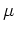and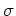is defined. Similarly, by assuming that the diffusion approximation holds (that is, the odd moments are assumed to be small)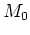and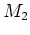fix a distribution function in three dimensions, which gives the relation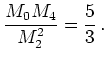(3.18)

Hence, naturally, the assumption of gaussianity gives a closure for the hierarchy of moment equations at order 4.

For probability distributions (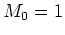) the kurtosis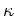is defined as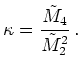(3.19)

Note that here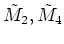denote the central moments of a one-dimensional probability distribution. The kurtosis is one invariant of the class of Maxwellian distributions. Deviations from this constant value are measures of nongaussianity.

Other invariants exist and can be used to define closure relations. One such family of invariants was used in [GKGS01] to express the sixth moment as a function of the lower moments.

Similar to the kurtosis is the dimensionless quantity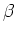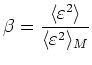(3.20)

Here the subscript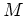denotes the Maxwellian distribution with local temperature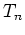. In terms of momentsis given by Equation 3.14.

Then we approximate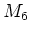by ([Gri02], p.24)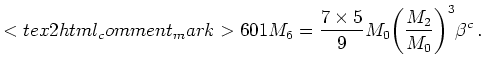(3.21)

In terms of moments this closure is written as(3.22)

For this type of closure the sixth moment is a rational function in the even moments. When this is combined with the use of mobilities to describe the scattering integral this method can be easily implemented by extending an existing drift-diffusion code.

A suitable value of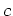is found by comparison with Monte Carlo data. This approach was developed in [GKGS01], [Gri02], wherewas assumed integer.

Forwe getfrom a Maxwellian with temperatureby the relation: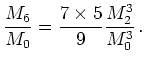(3.23)

For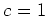a dimensionless parameter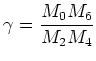(3.24)

can be introduced. The closure becomes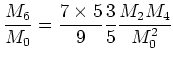(3.25)

This closure was studied in [SYT+96].

Forwe get another Gaussian invariant and introduce a parameter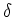as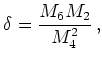(3.26)

which is (up to a constant factor) the quotient between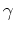and. The definition ofis a generalization of the kurtosis. This gives the closure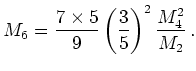(3.27)

Note that only for this value ofthe closure does not depend on.

Finally, for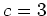we get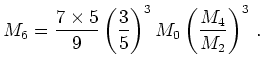(3.28)

It is only for the value ofthat the sixth moment goes with the first power of.

In general the given moments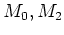, andcannot be represented as moments of a Gaussian distribution. Yet all closures of the family impose some type of gaussianity condition on the moments of the distribution function.

As the generalized invariant approach does not make an ansatz for the distribution function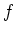it has the advantage that the domain of skewness and kurtosis which can be represented is in principle not restricted, which improves the fourth order closure 3.18.